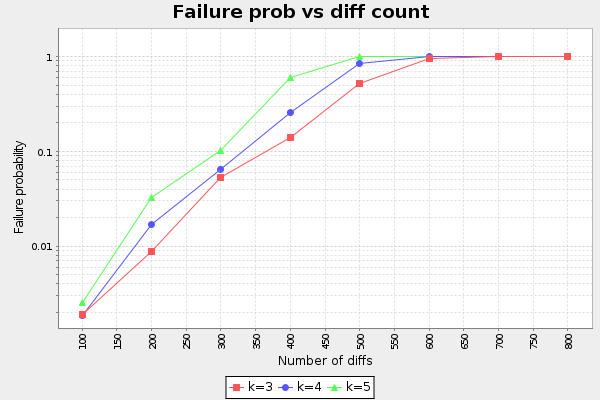IBLT Part IV: Increasing differences

<< Part III | This is Part IV of a multi-part series on Bitcoin block propagation with IBLT. | Part V >>

Failure probability will increase when we squeeze more differences into the IBLT. Basically, I want to answer the following question:

How will failure probability increase when differences increase?

So, with a fixed IBLT, how will failure probability grow with increasing differences? We select an IBLT with 3000 cells. We then try to encode and decode 100, 200, 300, .., 800 differences. In each step we run enough samples (1000, 10000 or 100000) to establish the failure probability. Also, to further establish that k=3 is the best choice for number of hash functions, the process was repeated for k=3, k=4 and k=5. In Part II we only tested for minimum decodable IBLT (Failure probability close to 1). Here we will test k for different failure probabilities.

Ladies and gentlemen, we have a result:We can observe at least two things:

1. k=3 is still the best choice.
2. The graph is pretty much linear with log(p)

Observation 1 was what we expected. Good!

Observation 2 means that failure probability grows with about O(A^d) for some constant A and number of differences d. For k=3, A is somewhere around 1.014. The means that doubling d will increase the failure probability by a factor A^diffs. For example, when d=200 and p=0.009, if we double the diffs to 400 we will get failure probability 0.009*1.014^200=0.015. The approximation is: